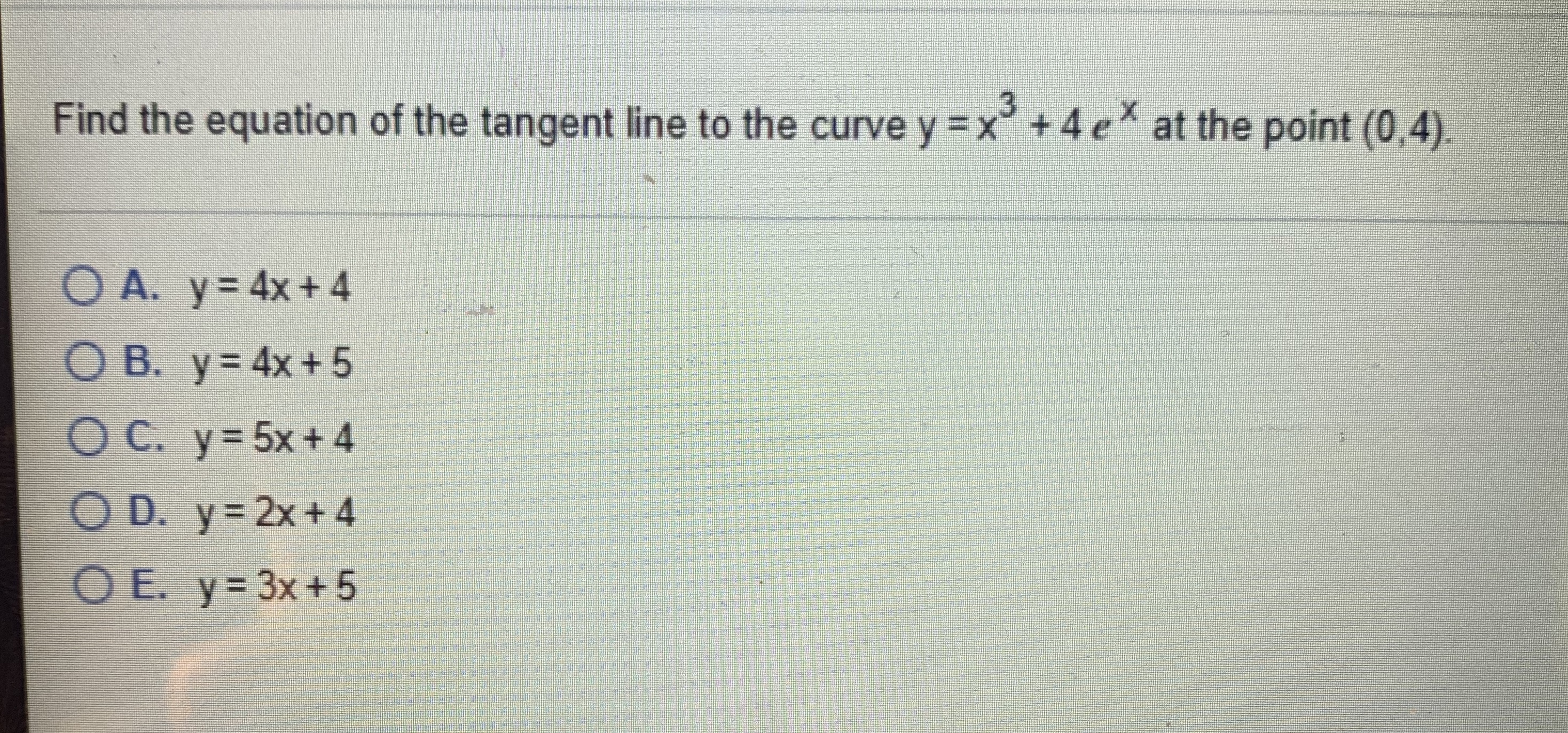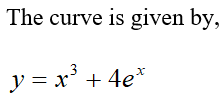# Find the equation of the tangent line to the curve y =x° +4 eat the point (0,4).O A. y= 4x+4O B. y= 4x+ 5OC. y=5x+4O D. y= 2x+4OE. y= 3x+5

Question
1 views

I attached a picture of the questionhelp_outlineImage TranscriptioncloseFind the equation of the tangent line to the curve y =x° +4 e at the point (0,4). O A. y= 4x+4 O B. y= 4x+ 5 OC. y=5x+4 O D. y= 2x+4 OE. y= 3x+5 fullscreen
check_circle

star
star
star
star
star
1 Rating
Step 1...

### Want to see the full answer?

See Solution

#### Want to see this answer and more?

Solutions are written by subject experts who are available 24/7. Questions are typically answered within 1 hour.*

See Solution
*Response times may vary by subject and question.
Tagged in